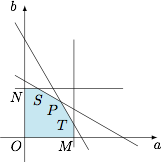# 每日一题约束条件下的最值

（2）当 $(a,b)$ 在第 $(1)$ 小题所得的约束条件内移动时，求 $\triangle ABC$ 面积的最大值，并求此时 $(a,b)$ 的值．其中 $M\left(1,0\right)$，$N\left(0,1\right)$，$P\left(\sqrt 3-1,\sqrt 3-1\right)$，$S\left(2-\sqrt 3,1\right)$，$T\left(1,2-\sqrt3\right)$．

（2）考虑到$\begin{split} &OM^2=ON^2=1,\\&OT^2=OS^2=OP^2=8-4\sqrt 3,\end{split}$于是 $\triangle ABC$ 面积的最大值为$\dfrac{\sqrt 3}4\cdot \left(8-4\sqrt 3\right)=2\sqrt 3-3,$此时 $(a,b)=\left(1,2-\sqrt 3\right),\left(2-\sqrt 3,1\right),\left(\sqrt 3-1,\sqrt 3-1\right)$．